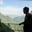Related Tags

python
communitycreator
scatter plot

# How to draw a scatter plot using Seaborn in PythonAKASH BAJWA

A scatter plot displays data between two continuous data. It shows how one data variable affects the other variable. A scatter plot can display data in different types of plots, both 2D and 3D.

Seaborn is a widely-used library in Python for data visualization. It represents data in a straightforward way in the form of plots.

Seaborn offers different ways of styling the plots, such as by changing the color palette with multiple options. In Seaborn, we use the scatterplot() method to create plots.

### Syntax

sns.scatterplot(x, y, data)

sns: It is the Seaborn variable.

### Parameters

• x: It is the data value on the x-axis.
• y: It is the data value on the y-axis.
• data: It is a DataFrame containing variables and observations.

### Code

In the code snippet below, we visualize a data frame in pictorial form:

import pandas as pd
import seaborn as sns
import matplotlib.pyplot as plt
# A list containing year values
Year = [2000,2001,2002,2003,2004,2005,2006,
2007,2008,2009,2010,2011,2012,2013,2014,2015,2016]
# A list containing profit values
Profit = [90, 65.8, 74, 65, 99.5, 19,
33.6,23,35,12,86,34,867,20,70,64,44]
# pd.DataFrame converts lists to a dataframe
data_plot = pd.DataFrame({"Year":Year, "Profit":Profit})
# the scatterplot function  represent data in the form of dots
sns.scatterplot(x = "Year", y = "Profit", data= data_plot)

### Code explanation

• Line 5: We create a list named Year to include the years.
• Line 8: We create a list named Profit to include the profit figures.
• Line 11: We create a data frame with Year as the x-axis label and Profit as the y-axis label.
• Line 13: We invoke the sns.scatterplot() function from the Seaborn library to generate a scatter plot on the data above.

RELATED TAGS

python
communitycreator
scatter plot

CONTRIBUTORAKASH BAJWA
RELATED COURSES

View all Courses

Keep Exploring

Learn in-demand tech skills in half the time